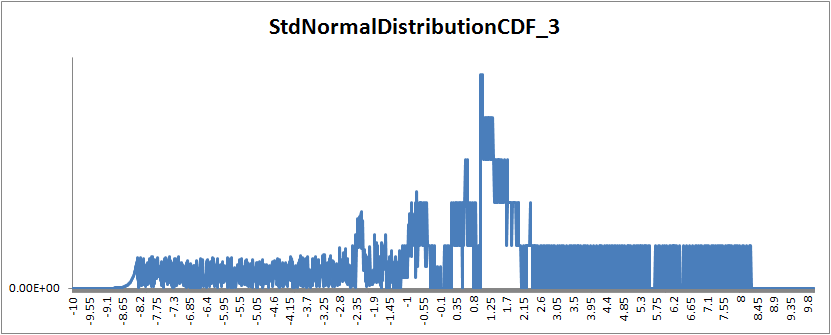12 September 2012

Part 5: T-SQL Implementation of NORMDIST / NORM.S.DIST

This is Part 5 of a set of posts. The other posts are:
Part 1: Introduction
Part 2: Probability Density Function Implementation
Part 3: Cumulative Distribution Function Implementation 1
Part 4: Cumulative Distribution Function Implementation 2
Part 5: Cumulative Distribution Function Implementation 3
Part 6: References and Notes

Cumulative Distribution Function Implementation 3

The cumulative distribution function has no closed form expression and can only be approximated (see Part 1.)
The following implementation uses a set of rational approximations from W. J. Cody (1969) (see Part 6 for full references.)

-- =============================================
-- Author:    Eli Algranti
-- Description:  Standard Normal Distribution
--        Cumulative Distribution Function
--        using a rational polynomial
--        approximation to erf() from
--        W. J. Cody 1969
-- Copyright:  Eli Algranti (c) 2012
--
-- This code is licensed under the Microsoft Public
-- =============================================
CREATE FUNCTION [dbo].[StdNormalDistributionCDF_3] ( @x FLOAT)
RETURNS FLOAT
AS
BEGIN
DECLARE @Z FLOAT = ABS(@x)/SQRT(2.0);
DECLARE @Z2 FLOAT = @Z*@Z; -- optimization

IF (@Z >=11.0) -- value is too large no need to compute
BEGIN
IF @x > 0.0
RETURN 1.0;
RETURN 0.0;
END

-- Compute ERF using W. J. Cody 1969

DECLARE @ERF FLOAT;

IF (@Z <= 0.46786)
BEGIN
DECLARE @pA0 FLOAT = 3.209377589138469472562E03;
DECLARE @pA1 FLOAT = 3.774852376853020208137E02;
DECLARE @pA2 FLOAT = 1.138641541510501556495E02;
DECLARE @pA3 FLOAT = 3.161123743870565596947E00;
DECLARE @pA4 FLOAT = 1.857777061846031526730E-01;

DECLARE @qA0 FLOAT = 2.844236833439170622273E03;
DECLARE @qA1 FLOAT = 1.282616526077372275645E03;
DECLARE @qA2 FLOAT = 2.440246379344441733056E02;
DECLARE @qA3 FLOAT = 2.360129095234412093499E01;
DECLARE @qA4 FLOAT = 1.000000000000000000000E00;

-- For efficiency compute sequence of powers of @Z
-- (instead of calling POWER(@Z,2), POWER(@Z,4), etc.)
DECLARE @ZA4 FLOAT = @Z2*@Z2;
DECLARE @ZA6 FLOAT = @ZA4*@Z2;
DECLARE @ZA8 FLOAT = @ZA6*@Z2;

SELECT @ERF = @Z *
(@pA0 + @pA1*@Z2 + @pA2*@ZA4 + @pA3*@ZA6 + @pA4*@ZA8) /
(@qA0 + @qA1*@Z2 + @qA2*@ZA4 + @qA3*@ZA6 + @qA4*@ZA8);
END
ELSE IF (@Z <= 4.0)
BEGIN
DECLARE @pB0 FLOAT = 1.23033935479799725272E03;
DECLARE @pB1 FLOAT = 2.05107837782607146532E03;
DECLARE @pB2 FLOAT = 1.71204761263407058314E03;
DECLARE @pB3 FLOAT = 8.81952221241769090411E02;
DECLARE @pB4 FLOAT = 2.98635138197400131132E02;
DECLARE @pB5 FLOAT = 6.61191906371416294775E01;
DECLARE @pB6 FLOAT = 8.88314979438837594118E00;
DECLARE @pB7 FLOAT = 5.64188496988670089180E-01;
DECLARE @pB8 FLOAT = 2.15311535474403846343E-08;

DECLARE @qB0 FLOAT = 1.23033935480374942043E03;
DECLARE @qB1 FLOAT = 3.43936767414372163696E03;
DECLARE @qB2 FLOAT = 4.36261909014324715820E03;
DECLARE @qB3 FLOAT = 3.29079923573345962678E03;
DECLARE @qB4 FLOAT = 1.62138957456669018874E03;
DECLARE @qB5 FLOAT = 5.37181101862009857509E02;
DECLARE @qB6 FLOAT = 1.17693950891312499305E02;
DECLARE @qB7 FLOAT = 1.57449261107098347253E01;
DECLARE @qB8 FLOAT = 1.00000000000000000000E00;

-- For efficiency compute sequence of powers of @Z
-- (instead of calling POWER(@Z,2), POWER(@Z,3), etc.)
DECLARE @ZB3 FLOAT = @Z2*@Z;
DECLARE @ZB4 FLOAT = @ZB3*@Z;
DECLARE @ZB5 FLOAT = @ZB4*@Z;
DECLARE @ZB6 FLOAT = @ZB5*@Z;
DECLARE @ZB7 FLOAT = @ZB6*@Z;
DECLARE @ZB8 FLOAT = @ZB7*@Z;

SELECT @ERF = 1.0 - EXP(-@Z2) *
(@pB0 + @pB1*@Z + @pB2*@Z2 + @pB3*@ZB3 + @pB4*@ZB4
+ @pB5*@ZB5 + @pB6*@ZB6 + @pB7*@ZB7 + @pB8*@ZB8) /
(@qB0 + @qB1*@Z + @qB2*@Z2 + @qB3*@ZB3 + @qB4*@ZB4
+ @qB5*@ZB5 + @qB6*@ZB6 + @qB7*@ZB7 + @qB8*@ZB8);
END
ELSE
BEGIN
DECLARE @pC0 FLOAT = -6.58749161529837803157E-04;
DECLARE @pC1 FLOAT = -1.60837851487422766278E-02;
DECLARE @pC2 FLOAT = -1.25781726111229246204E-01;
DECLARE @pC3 FLOAT = -3.60344899949804439429E-01;
DECLARE @pC4 FLOAT = -3.05326634961232344035E-01;
DECLARE @pC5 FLOAT = -1.63153871373020978498E-02;

DECLARE @qC0 FLOAT = 2.33520497626869185443E-03;
DECLARE @qC1 FLOAT = 6.05183413124413191178E-02;
DECLARE @qC2 FLOAT = 5.27905102951428412248E-01;
DECLARE @qC3 FLOAT = 1.87295284992346047209E00;
DECLARE @qC4 FLOAT = 2.56852019228982242072E00;
DECLARE @qC5 FLOAT = 1.00000000000000000000E00;

DECLARE @pi FLOAT = 3.141592653589793238462643383;

-- For efficiency compute sequence of powers of @Z
-- (instead of calling POWER(@Z,-2), POWER(@Z,-3), etc.)
DECLARE @ZC2 FLOAT = (1/@Z)/@Z;
DECLARE @ZC4 FLOAT = @ZC2*@ZC2;
DECLARE @ZC6 FLOAT = @ZC4*@ZC2;
DECLARE @ZC8 FLOAT = @ZC6*@ZC2;
DECLARE @ZC10 FLOAT = @ZC8*@ZC2;

SELECT @ERF = 1 - EXP(-@Z2)/@Z * (1/SQRT(@pi) + 1/(@Z2)*
((@pC0 + @pC1*@ZC2 + @pC2*@ZC4 + @pC3*@ZC6 + @pC4*@ZC8 + @pC5*@ZC10) /
(@qC0 + @qC1*@ZC2 + @qC2*@ZC4 + @qC3*@ZC6 + @qC4*@ZC8 + @qC5*@ZC10)));
END

DECLARE @cd FLOAT = 0.5*(1+@ERF);

IF @x > 0
RETURN @cd;

RETURN 1.0-@cd;
END

Verification Using Excel 2010 as Reference

Maximum difference 5.55111512312578E-16 at value 1.00999999999983Verification Using Excel 2007 as Reference

Maximum difference 5.09148279093097E-13 at value 4.97999999999994Discussion

This more complex and slower implementation uses three different rational approximations to provide maximum accuracy over a wide range of values.
Here at last we see the differences between Excel 2010 and Excel 2007.
Using Excel 2010 as reference we note the approximation provides the maximum precision possible with a double precision float (15.5 decimal places.)
Using Excel 2007 the precision drops down to 12 decimal places. The graph shows this happens for only two small bands at around 5 and -5 which is where the Excel 2007 implementation is lacking and where Excel 2010 shows improvements.

1.1.